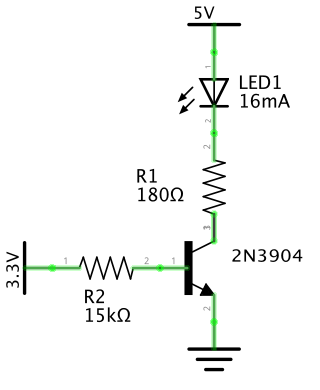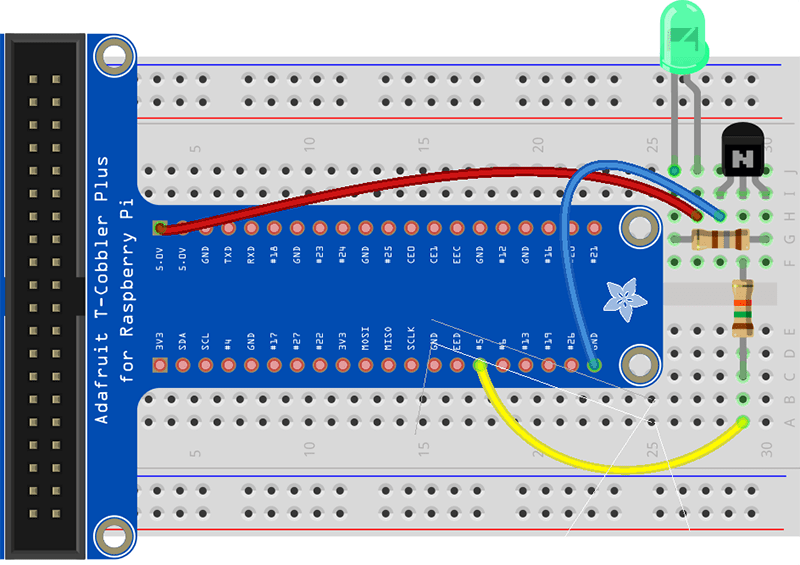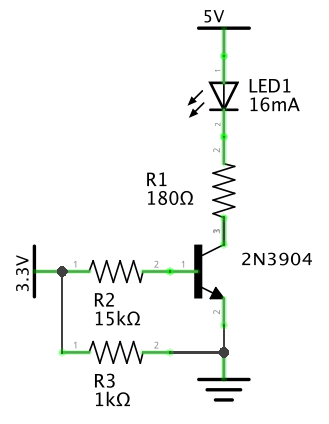# Putting the GPIO pin behind transistor to get more current

## In this example we are going to let the GPIO pin control a transistor so that we can draw more power when controlling a LED than what the GPIO pin can supply.

We will be using a simple LED in this example where we aim to draw 16 mA power (I could easily go higher using this method if I had bigger LED that can draw more power since the current will mostly come from the 5V and not from the GPIO pin it self), load on the GPIO pin will only be around 0,00018 A.

At far bottom of this article we will also deal with the initial undetermined state of the GPIO pin.

A transistor is basically a switch that is triggered by tiny electric signal. (See here how to understand transistor specification sheets)

Transistor is suitable to use when switching within the same circuit. While if the power your switching is in completely different circuit then you would want to use a optocoupler which is another kind of electric switch where only light signal is sent between the two circuits keeping them isolated. (See our guide about Optocouplers)

There are NPN and PNP transistors. The NPN ones switch on the low side (-) while PNP transistors switch on the high side (+). This is important difference and for example this difference would mean that the NPN transistor that we will be using in this example would not have been suitable for the RGB LED example since there we would need to switch on the high side (+) as the RGB LED had 3 anodes (+) and only one cathode (-). In the RGB LED example the LED was not shining very bright at all since we could not provide a lot of power from the GPIO pin, a transistor solves such issues.

Disclaimer:
We do not take any responsibility for possible errors in the guide or errors that you might do wiring it up. Incorrect wiring can result in damaged sensor or damaged Raspberry PI.

##### Pieces we use are:
• Raspberry PI 2
• Cobbler and Cobbler cable
• Green LED (can be any LED you want)
• 2N3904 Transistor (This one is common generic use NPN transistor, which you can easily substitute with many other such as BC548 and others). Their really cheap, I paid \$1.22 for 100 pieces in store called Electronic Gadget World on Ali Express. This included free shipping to Iceland. Thats 1,2 cents per transistor.
• Some resistors (types depend on specs of your RGB led and your transistor)
• Wiring PI Xojo module from Paul Lefebvre

If you don’t know resistor color codings to find correct resistors then you can click the link bellow

##### Drawing up the circuit:

First we just draw up the circuit without knowing the values of the resistors.Here is what we know:

• GPIO pin is 3,3 V
• The power we will be using from the Raspberry Power pins is 5 V
• The led I had was rated 1,9 – 2,5 V and 5 mA – 17,5 mA, (I will be pushing it to about 16 mA at 2,2 V)
• The transistor I had was 2N3904 which is NPN transistor. (NPN transistors usually need around 0,7 V for its base)

Now we calculate the rest:

• R1 would be calculated as Ohms law, R = V / I
(5V – 2,2V) / 0,016 A = 175 Ω   (We will use 180 Ω)
5 – 2,2 is because the LED will use 2,2 so we have to let the resistor take the rest of the 5 V which means resistor will be at 2,8 V.
• For R2 then the following applies:
1. We know the Base of the transistor wants around 0,7 V since that is  what NPN transistors usually want. So the voltage over R2 has to be 3,3V – 0,7V = 2,6V.
2. For the current then we need to apply the following formula: I(B) = I(C) / β.  (The β will usually be marked as hfc in the transistors specs) You google the spec for your transistor and find the Beta Value, it will have some range depending on current, it will be marked as On characteristics in the spec. Mine has the β spec of 100 to 300 at 10 mA and 60 to 300 at 50 mA. Since we are using 16 mA then we will choose β of 90.
Which means I(B) will be: 0,016 / 90 = 0,00018 A (This is how little we will be taking from the GPIO pin)

Now that we have the voltage and current then R2 = 2,6 V / 0,00018 A = 14,4 kΩ  (We will use 15 kΩ resistor for R2)

We we update our circuit wit the calculated values of R1 and R2:We will use GPIO 5 for the GPIO pin.

For the led then you can see in the picture bellow then that you have two ways to identify the + and – or anode vs cathode. Its the length of the pins where the longer wire is the anode and the shorter is the cathode. If wires have been trimmed then you can usually also see it on the LED it self the flat side on the edge is the cathode or – as is shown on the picture to right here.When looking up the spec for your transistor then the spec will usually show how the pin layout is so you can know which was it should turn.

For mine it was like this:When putting the parts on the breadboard you should try to go by the circuit drawing instead of blindly following the breadboard drawing since its easier to make mistakes if not understanding the wiring.

If you are not familiar with breadboards and placement of the cobbler then check this link out.# The Xojo code for this one really simple as this was more of electric guide than coding guide

We just had one button on a window with the caption “Toggle LED”.

In the window I added the following property:

`Protected ledOn As Boolean = false`

The Window Open event was as follows:

The open event of the window:

```Sub Open()
GPIO.SetupGPIO
GPIO.PinMode(5,GPIO.OUTPUT)
GPIO.DigitalWrite(5,GPIO.OFF)
End Sub```

The button box event:

```Sub Action()
if ledOn then
GPIO.DigitalWrite(5,GPIO.OFF)
ledOn = false
else
GPIO.DigitalWrite(5,GPIO.ON)
ledOn = true
end if
End Sub```

#### Un determined state on the GPIO pin at startup

Now I was not 100% happy when running it, the LED was on when I booted the computer which is caused by the GPIO pin having undetermined state.

Everything else worked, the code was able to turn it on and off, and the LED was shining bright as it was not having to be conservative on the current it takes.

There was easy fix to that, adding 1kΩ resistor between the GPIO pin and the ground.

Making the circuit like this:After adding the 1kΩ resistor then the LED was shut when batting the computer and it only got turned on and off when sending the signal from the program.

Thats is for now.

```AliExpress.com Product - HoldPeak HP-770D Multimeter ```
```AliExpress.com Product - 15Values TO-92 Transistor Assorted Kit S9012 S9013 S9014 S8050 S8550 2N3904 2N3906 BC327 BC337 Tl431 A42 A92 A1015 C1815 13001 ```